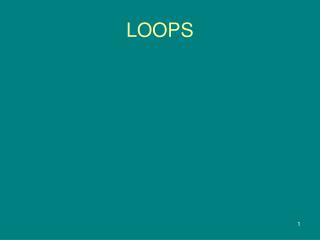DownloadDownload PresentationLOOPS

# LOOPS

Download Presentation## LOOPS

- - - - - - - - - - - - - - - - - - - - - - - - - - - E N D - - - - - - - - - - - - - - - - - - - - - - - - - - -
##### Presentation Transcript

1. LOOPS

2. Repetitions • while Loops • do-while Loops • for Loops • break and continue

3. while Loop Flow Chart int count = 0; while (count < 100) { System.out.println("Welcome to Java!"); count++; } while (loop-continuation-condition) { // loop-body; Statement(s); }

4. Example 3.2: Using while Loops Problem: Write a program that reads and calculates the sum of an unspecified number of integers. The input 0 signifies the end of the input.

5. Caution Don’t use floating-point values for equality checking in a loop control. Since floating-point values are approximations, using them could result in imprecise counter values and inaccurate results. This example uses int value for data. If a floating-point type value is used for data, (data != 0) may be true even though data is 0. // data should be zero double data = Math.pow(Math.sqrt(2), 2) - 2; if (data == 0) System.out.println("data is zero"); else System.out.println("data is not zero");

6. do-while Loop do { // Loop body; Statement(s); } while (loop-continuation-condition);

7. for Loops int i; for (i = 0; i < 100; i++) { System.out.println( "Welcome to Java!"); } for (initial-action; loop-continuation-condition; action-after-each-iteration) { // loop body; Statement(s); }

8. Note • The initial-action in a for loop can be a list of zero or more comma-separated expressions. The action-after-each-iteration in a for loop can be a list of zero or more comma-separated statements. Therefore, the following two for loops are correct. They are rarely used in practice, however. • for (int i = 1; i < 100; System.out.println(i++)); • for (int i = 0, j = 0; (i + j < 10); i++, j++) { • // Do something • }

9. Note If the loop-continuation-condition in a for loop is omitted, it is implicitly true. Thus the statement given below in (A), which is an infinite loop, is correct. Nevertheless, I recommend that you use the equivalent loop in (B) to avoid confusion:

10. Which Loop to Use? The three forms of loop statements, while, do-while, and for, are expressively equivalent; that is, you can write a loop in any of these three forms.For example, a while loop in (A) in the following figure can always be converted into the following for loop in (B): A for loop in (A) in the following figure can generally be converted into the following while loop in (B) except in certain special cases (see Review Question 3.19 for one of them):

11. Recommendations I recommend that you use the one that is most intuitive and comfortable for you. In general, a for loop may be used if the number of repetitions is known, as, for example, when you need to print a message 100 times. A while loop may be used if the number of repetitions is not known, as in the case of reading the numbers until the input is 0. A do-while loop can be used to replace a while loop if the loop body has to be executed before testing the continuation condition.

12. Caution Adding a semicolon at the end of the for clause before the loop body is a common mistake, as shown below: for (int i=0; i<10; i++); { System.out.println("i is " + i); } Logic Error

13. Caution, cont. Logic Error Similarly, the following loop is also wrong: int i=0; while (i < 10); { System.out.println("i is " + i); i++; } In the case of the do loop, the following semicolon is needed to end the loop. int i=0; do { System.out.println("i is " + i); i++; } while (i<10); Correct

14. Using the Keywords break and continue

15. The continue Keyword

16. Example 3.8 Finding the Sales Amount • Problem: You have just started a sales job in a department store. Your pay consists of a base salary and a commission. The base salary is \$5,000. The scheme shown below is used to determine the commission rate. • Sales Amount Commission Rate • \$0.01–\$5,000 8 percent • \$5,000.01–\$10,000 10 percent • \$10,000.01 and above 12 percent • Your goal is to earn \$30,000 in a year. Write a program that will find out the minimum amount of sales you have to generate in order to make \$30,000.